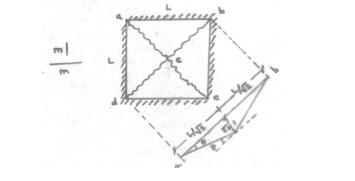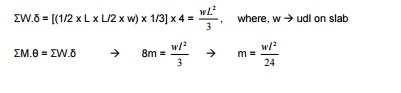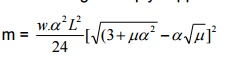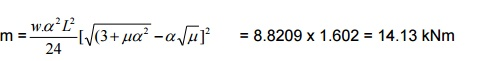# Yield Line TheoryApplication of virtual work method to square, rectangular, circular and triangular slabs

YIELD LINE THEORY

Yield lines -Typical crack patterns -generated when ultimate moment is reached Characteristics of yield lines are,

i) Yield lines are straight

ii) Yield lines end at supporting edges of slab

iii) Yield lines passes through intersection of axis of rotation of adjacent slab elements

Axis of rotation lies along lines of supports and passes over columnsAssumptions:

The following are the assumptions of the yield line analysis of reinforced concrete slabs.

1.  The steel reinforcement is fully yielded along the yield lines at collapse. Rotation following yield is at constant moment.

2.The slab deforms plastically at collapse and is separated into segments by the yield lines. The individual segments of the slab behave elastically.

3.  The elastic deformations are neglected and plastic deformations are only considered. The entire deformations, therefore, take place only along the yield lines. The individual segments of the slab remain plane even in the collapse condition.

4.  The bending and twisting moments are uniformly distributed along the yield lines. The maximum values of the moments depend on the capacities of the section based on the amount of reinforcement provided in the section.

5. The yield lines are straight lines as they are the lines of intersection between two planes.

Rules of yield lines:

The two terms, positive and negative yield lines, are used in the analysis to designate the yield lines for positive bending moments having tension at the bottom and negative bending moments having tension at the top of the slab, respectively.

The following are the guidelines for predicting the yield lines and axes of rotation:

1. Yield lines between two intersecting planes are straight lines.

2. Positive yield line will be at the mid-span of one-way simply supported slabs.

3. Negative yield lines will occur at the supports in addition to the positive yield lines at the mid-span of one-way continuous slabs.

4. Yield lines will occur under point loads and they will be radiating outward from the point of application of the point loads.

5. Yield line between two slab segments should pass through the point of intersection of the axes of rotation of the adjacent slab segments.

6. Yield lines should end at the boundary of the slab or at another yield line.

7. Yield lines represent the axes of rotation.

8. Supported edges of the slab will also act as axes of rotation. However, the fixed supports provide constant resistance to rotation having negative yield lines at the supported edges. On the other hand, axes of rotation at the simply supported edges will not provide any resistance to rotation of the segment.

Axis of rotation will pass over any column support, if provided, whose orientation will depend on other considerations.

Upper and Lower Bound Theorems

According to the general theory of structural plasticity, the collapse load of a structure lies in between the upper bound and lower bound of the true collapse load. Therefore, the solution employing the theory of plasticity should ensure that lower and upper bounds converge to the unique and correct values of the collapse load.

The statements of the two theorems applied to slabs are given below:

(A) Lower bound theorem: The lower bound of the true collapse load is that external load for which a distribution of moments can be found satisfying the requirements of equilibrium and boundary conditions so that the moments at any location do not exceed the yield moment.

(B) Upper bound theorem: The upper bound of the true collapse load is that external load for which the internal work done by the slab for a small increment of displacement, assuming that moment at every plastic hinge is equal to the yield moment and satisfying the boundary conditions, is equal to the external work done by that external load for the same amount of small increment of displacement.

Thus, the collapse load satisfying the lower bound theorem is always lower than or equal to the true collapse load. On the other hand, the collapse load satisfying the upper bound theorem is always higher than or equal to the true collapse load.

The yield line analysis is an upper bound method in which the predicted failure load of a slab for given moment of resistance (capacity) may be higher than the true value. Thus, the solution of the upper bound method (yield line analysis) may result into unsafe design if the lowest mechanism could not be chosen. However, it has been observed that the prediction of the most probable true mechanism in slab is not difficult. Thus, the solution is safe and adequate in most of the cases. However, it is always desirable to employ a lower bound method, which is totally safe from the design point of view.1. Virtual work method

2. Equilibrium method (Equilibrium of individual elements of slab along yield line)

Virtual work method -Applied load causing virtual displacement is equal to internal work done or energy dissipated in rotation along the yield lines.

I) Isotropically reinforced -square slab -simply supported -udl

II) Isotropically reinforced -square slab -fixed on all edges -udl

III) Orthotropically reinforced (Diff. rein. bothways) -rectangular slab -simply supported -udl

IV) Orthotropically reinforced -rectangular slab -fixed along long edges - simply supported along short edges -udl

V) Orthotropically reinforced -rectangular slab -all four edges fixed -udl

I) Isotropically reinforced -square slab -simply supported -udl

External work done = w.?Where, w load,virtual? displacement

Internal work done = M.? = ?m.L.?

m Ultimate moment / unit length

L Length of yield line

M Total moment produced along all the yield lines[Opposite of isotropically reinforced is orthotropically reinforced]

Centre point is the place where the first ultimate moment is reached and the crack originates at this point as,

Work done by �bd? is same as �ac? Total internal work done = ?M.? = 8m

For a virtual displacement of 1 at centre (i.e) CG of each triangular deflects 1/3II) Isotropically reinforced -square slab -fixed on all edges -udlIII) Orthotropically reinforced (Diff. rein. bothways) -rectangular slab -simply supported -udlIV) Orthotropically reinforced -rectangular slab -fixed along long edges - simply supported along short edges -udlV) Orthotropically reinforced -rectangular slab -all four edges fixed -udl1.Design a square slab fixed along all four edges, which is of side 5m. The slab has to support a service load of 4kN/m2. Use M20 concrete and Fe415 steel.

As per IS 456-2000,

l/d = (0.8 x 35) = 28 5000 / 28 = d

d = 178.6mm = 180mm

Provide D = 200mm

Self weight = 0.2 x 25 = 5 kN/m2

Floor finish = 1 kN/m2

Total = 10 kN/m2

Factored load (wu) = 1.5 x 10 = 15 kN/m2

By yield line theory, m = wL2 / 48 = =7.8125kNm

Limiting moment, Mulim = 0.138.fck.b.d2 = 0.138 x 20 x 1000 x 1802 = 89.424 x 106 Nmm Mu < Mulim

K = Mu/bd2 = 0.241

Ast = 122mm2

Provide 8mm @ 300mm c/c

1b) In the above problem, design the slab using IS456 coefficient method.

ly / lx = 1

Four edges are discontinuous ?x = y ?=0.056

Mx = x.?w.lx2 = 0.056 x 15 x 52 = 21 kNm My = y.?w.lx2 = 0.056 x 15 x 52 = 21 kNm

Required spacing of 8mm bar is 140mm. Provide 8mm @ 140mm c/c.

2. Design a square slab of size 5m, simply supported along its four edges and subjected to a live load of 4kN/m2.

m =wL2/24

As per IS 456-2000,

l/d = (0.8 x 35) = 28 5000 / 28 = d

d = 178.6mm = 180mm

Provide D = 200mm

Self weight = 0.2 x 25   = 5 kN/m2

Floor finish                                       = 1 kN/m2

Total           = 10 kN/m2

Factored load (wu) = 1.5 x 10 = 15 kN/m2

By yield line theory,      m  =  wL2/24        = 15.625kNm

Limiting moment, Mulim = 0.138.fck.b.d2 = 0.138 x 20 x 1000 x 1802 = 89.424 x 106 Nmm Mu < Mulim

Ast = 247.59mm2

Provide 8mm @ 200mm c/c

3. Design a rectangular slab of size 4m x 6m simply supported along all its edges, subjected to a live load of 4kN/m2. The coefficient of orthotrophy is 0.7. Use M20 and Fe415.

For four edges simply supported condition,Assume span/depth = 28 Eff.depth = 4000/28 = 142.86mm

Provide d = 150mm, D = 170mm

Self weight = 0.17 x 25 = 4.25 kN/m2

Floor finish = = 0.75 kN/m2

Total = 9 kN/m2

Factored load (wu) = 1.5 x 9 = 13.5 kN/m2Limiting moment, Mulim = 0.138.fck.b.d2 = 62.1 kNm

Mu < Mulim. The section is under-reinforced.

K = Mu/b.d2 Ast = 277mm2

Minimum Ast = 0.12% of c/s = 204mm2

Ast along   longerst = span0.7x237 ==194mm? 2x< 204A mm2

Provide 8mm @ 240mm c/c

Check for shear (factored):?c for [100Ast/bd = (100 x 277)/(1000 x 150) =

0.185N/mm2] 0.15 0.28

0.25 0.36

0.185 0.308

As per Cl.42.4 of IS456-2000,

K 1.3 for d = 150mm

K 1 for d = 300mm

K ((1.126 + 0.133) = 1.26) for d = 170mm

K. ?c= 1.26 x 0.308 = 0.388 N/mm2 > v (0?.18 N/mm2)

Section is safe in shear.

4.In the above problem, design the slab if all the supports are fixed. For four edges simply supported condition,

K. ?c= 1.26 x 0.308 = 0.388 N/mm2 > v (0?.18 N/mm2)

Section is safe in shear.5. Solve the above problem if two long edges are fixed.

Provide 8mm @ 240mm bothways.Triangular slab:

I)             Isotropically reinforced -Equilateral triangular slab -simply supported along all edges - udl

II)           Isotropically reinforced -Equilateral triangular slab -simply supported along two adjacent edges -udl

III)            III) Isotropically reinforced -Right angled triangular slab -simply supported along all edges -udl

IV) Isotropically reinforced -Circular slab -simply supported along edges -udl  ailure takes place by formation of infinite number of positive yield lines running radially  om centre to circumference, forming a flat cone at collapse.

6. Design an equilateral triangular slab of side 5m, isotropically reinforced and is simply supported along its edges. The slab is subjected to a superimposed load of 3kN/m2. Use M20 concrete and Fe415 steel.

Assume span/depth = 28 Eff.depth = 5000/28 =

178.57mm Provide d = 180mm, D = 200mm

Self weight   = 0.2 x 25 = 5 kN/m2

Floor finish = 1 kN/m2

Total = = 9 kN/m2

Factored load (wu) = 1.5 x 9 = 13.5 kN/m2

Limiting moment, Mulim = 0.138.fck.b.d2 = 89.42 kNm Mu < Mulim. The section is under-reinforced.

K = Mu/b.d2 Ast = 72.754mm2

Minimum Ast = 0.12% of c/s = 240mm2

Ast < min Ast [240 mm2]

Provide 8mm @ 200mm c/c

Check for shear (factored):0.185N/mm2

0.13 0.28

As per Cl.42.4 of IS456-2000,

K 1.3     for d = 150mm

K 1 for d = 300mm

K ((1.04 + 0.2) = 1.24) for d = 180mm

K. ?c= 1.24 x 0.28 = 0.3472 N/mm2 > v (0?.18 N/mm2)

Section is safe in shear.

Study Material, Lecturing Notes, Assignment, Reference, Wiki description explanation, brief detail
Civil : Design Of Reinforced Concrete And Brick Masonry Structures- Yield Line Theory : Yield Line Theory |